6.1 Introduction:

Geometry is that branch of mathematics which studies the properties of figures such us lines, triangles, quadrilaterals etc.

Ancient Indians used the geometrical properties for construction of fire places called ‘yajnakundas’. They also had profound knowledge of

astronomy, as even today the method of calculation of occurrences of eclipses are precise and date and times  are arrived at

many years in advance of their occurrence.

1.      Can you divide the below mentioned line segment in to two exact parts without using the scale?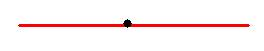2.      What should be the size of underground tank to store petrol/milk and water supplied through tankers?

3.      Why do people who prepare sweets, makers cut the ‘burfi’ in the shape of parallelograms rather than rectangulars?

In geometry we find answers/solutions to  above mentioned concepts/problems.

6.1.0 Definitions and some basic constructions:

Definition:

 ‘Angle’ is a figure formed by two rays with a common end point. They are measured in degrees (00 to 3600) The common end point is called ‘vertex’. In the above figure B is a vertex; ABC is an angle and is denoted by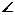ABC.  When we measure with protractor, we get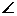ABC = 500. NoteABC =CBA = 500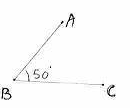No Type of angle Measure of angle is Example in the adjacent figure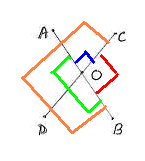1 Acute Angle Between 00 and 900AOC 2 Right Angle = 900 3 Obtuse Angle Between 900 and 1800 smallerCOB (clock wise direction) 4 Straight Angle = 1800( Angle on straight line)AOB 5 Reflex Angle Between 1800 and 3600 LargerBOC ( clock wise direction)

6.1.1. Construction of Perpendicular to a line from a point.

 Step 1: Draw the line AB of given measurement and C be an external point.   Step 2: With C as center, draw an arc of suitable radius to cut the line AB at 2 points (If necessary extend the line) X, Y as shown in the figure.   Step 3: With X and Y as centers, draw arcs (of radius more than half of XY) to cut at point Z on the other side of AB.   Step 4: Join C, Z to intersect the line AB at L   CL is perpendicular to AB.   Note: The procedure for construction of perpendicular at a point on the line is same as above. (C could be a point like L on AB)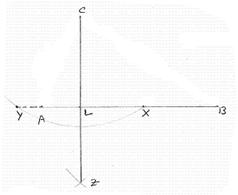Note:  Using SSS and SAS postulate (Refer Section 6.4.3), you can prove thatCLY =CLX = 900)

6.1.2. Construction of Perpendicular bisector to a line.

 Step1 : Draw the line AB of given measurement.   Step2: With A, B as centers, draw arcs of radius more than half the length of AB on both sides of AB. Let these arcs meet at X and Y.   Step3: Join XY to meet AB at L. (Note that XY bisects AB and XY is perpendicular to AB and L is mid point of AB)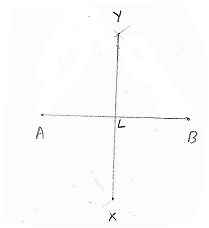Note:  Using SSS and then SAS postulate (Refer Section 6.4.3), you can prove that AL=BL andALY =YLB = 900)

6.1.3. Construction of Angular bisector.

 Step1: constructCAB of given measurement using protractor.   Step2: With A as center, draw arcs of same radius to cut the sides AB and AC at P and Q respectively.   Step3: With P and Q as centers as and with more than half of PQ as radius, draw arcs to intersect at R.   Step4: Join AR, AR is the angular bisector ofCAB. (CAR =RAB)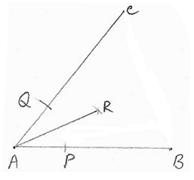Note:  Using SSS postulate (Refer Section 6.4.3), you can prove thatCAR =RAB)

 Locus is the path traced by a moving point, which moves so as to satisfy the given condition/conditions.   An example is the circumference of a circle.  Any point on a circumference is at equal distance from the center of the circle. Thus circumference is locus of circle.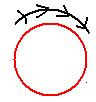6.1 Summary of learning

 No Points learnt 1 Definitions and basic constructions